# Challenging GRE QC Problems on Number Properties

Get your GRE math practice in with these nine GRE Quantitative Comparison questions, ranging in difficulty from moderate to quite hard (arguably as hard as, or perhaps harder than, anything you would see on the GRE Quant section).  Solutions will come at the end of the article.  Remember: don’t touch a calculator!

1)2)3)4) K is the set of perfect squares between 1 and 10000.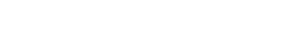5) When 900 is divided by positive integer D, the remainder is 1.  If N > 5000, then when N is divided by 23, the remainder is R.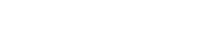6) F is the prime factorization of 17!7)8) N is a large integer, not divisible by 7.  When N is divided by 35, the quotient is Q1 and the remainder is R1. When N is divided by 7, the quotient is Q2 and the remainder is R2, and Q2 > 5Q1.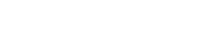9) The number N is any non-negative integer, and## Integer properties and fractions on the GRE QC

It’s important to remember that the GRE is not particularly interested in your ability to perform long detailed calculations.  In fact, if you find yourself getting sucked into a long detailed calculation anywhere on the GRE Quant section, you are probably doing something the hard way.  The GRE doesn’t care about long calculations, but it does care about your ability to use logic and number sense to simplify calculations.  It is interested in the test taker’s ability to re-frame the question in a way that leads to significant simplification.

Nowhere is this truer than it is on the Quantitative Comparisons.  Your job on the GRE QCs is to compare, not to calculate.  As a general rule, a GRE QC question is designed for a quite straightforward comparison or simplification.  It may appear to demand a long tedious calculation, but don’t be fooled: that is not the real issue.  The real issue is finding the way to look at the problem, the way to interpret it or reframe it, so that the pertinent comparison becomes much easier to see.

With fractions, think about simplifying comparisons: for example, bigger numerator makes the fraction bigger, but bigger denominator makes the fraction smaller.

With integer properties, there are a number of important distinctions that could come into play.  You should know what is what isn’t a prime number, as well as everything it means to be a prime number.  You should understand factors, multiples, prime factorizations, and remainders.

For difficult problems, as some of these are, it’s very important to read the solutions carefully.  Notice how the problem is framed in the solution, and notice what important observations were used to simplify it.## Practice problem solutions

1) This one isn’t too hard, but it can be simplified.  Notice that 6 is a multiple of 3 and 14 is a multiple of 7.  It’s much easier to find common denominators if one denominator is a multiple of the other, so group the fractions together accordingly.2) For this one, do NOT find common denominators.  It would be a nightmare to try to perform this exact calculation.  Instead, consider this perspective.  Look at the two fractions in Quantity A.  First, look at 10/23.  We know 10/20 = 1/2, so 10/23 is less than 1/2.  We also know that 19/38 = 1/2, so 19/37 is more than 1/2.  In Quantity A, we have
(something less than 1/2) minus (something greater than 1/2)

That has to be negative!  Quantity A is negative.  Meanwhile, we are adding two positive things in Quantity B, so that must be positive.  Any positive is greater than any negative.

3) These numbers are probably a little bit bigger than what you would be likely to see on the GRE, but notice that even for this, we don’t need a calculator!  Notice that both denominators are multiples of 25.

900 = 9*100 = 9*(4*25) = 36*25

500 = 5*100 = 5*(4*25) = 20*25

and, of course, 525 is just one more factor of 25, so

525 = 21*25

We can express both denominators in that factored form, and multiply both quantities by 25.Remember that cross-multiplying is a perfectly legitimate move in GRE QCs, as long as all the concerned numbers are positive.

4) Think about the numbers in K.  The first 10 perfect squares go from 1 to 100.  We know that 20 squared is 400 and 30 squared is 900, so the second set of ten perfect squares goes from 100 to 400, and the third set, from 400 to 900.  The spread is getting bigger.  We know that 90 squared is 8100 and 100 squared is 10,000, so the last set of ten is spread out between 8100 and 10000, a spread of almost 2000.

In other words, this set is clumped together at the low end and very spread out at the upper end.  The outliers are toward the high end.  The median is not affected by outliers, but the mean is pulled in the direction of outliers.   Therefore, the mean must be higher than the median.

That’s all you need to do to answer the question.  If you are interested in additional information: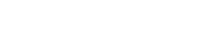The median is the average of those two numbers

median = 2550.5

I calculated the mean:

mean = 3383.5

There’s no particularly easy way to get that number without a calculator or computer.

5) Let’s think about integer D first.  D must be a factor of 900 – 1 = 899.  What are the factors of that number.  Well, we can think about this using the advanced numerical factoring, discussed in this GMAT blog.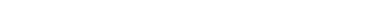Thus, 899 is the product of two prime numbers and, as such, has four factors: {1, 29, 31, 899}.  Well, if D = 1, then dividing any positive integer by it would never yield a remainder, so D can’t be 1.  D could be either 29 or 31 or 899.

Now, think about R.  When we divide by 23, the remainder must be less than 23.   Well, any number less than 23 will be less than any of the possibilities for D, so D is always bigger.

6) Consider the prime factorization of 17!—it must have many factors of 2 and 3.  It has a factor of 2 for every even number from 1 to 17, plus a second factor of 2 for every number divisible by 4, plus another for every number divisible by 8, etc.

By contrast, there are only three factors in 17! that contain factors of 5: {5, 10, 15}.  Each one contains exactly one factor of 5, so there are just three factors of 5 in 17! Quantity A = 3

The factors less than 5 have many more factors that 5 does.  The next prime number up is 7, which has two factors, from its multiples {7, 14}.  The next three prime factors are {11, 13, 17}, and each one of those is big enough no other multiples would be part of 17!  Thus, these three prime number, {11, 13, 17}, each have an exponent of one in F.  Quantity B = 3

7) To get started, think about smaller numbers.  How many multiples of 5 between 45 and 200?  Well, 45 = 5*9 and 200 = 5*40.  The number of multiples of 5 between these two is equivalent to the number of integers between 9 and 40.  If we subtract, 40 – 9 = 31, that excludes 9 but includes 40.  If we truly want the number of multiples between the two end points, I have to subtract one.  There are 30 multiples of 5 between 45 and 200.  Notice that, to find the number of multiples of 5, we simply divided the top and bottom number by 5 and subtracted then, then subtracted 1.  Follow this pattern.We will add 1 to both quantities, because it’s the same in both and it’s in the way.  In each quantity, we will factor out the lower power.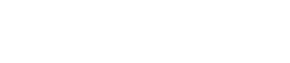Now, we can compare these part-wise.  First of all, of course 7 to the power of 10 must be larger than 3 to the power of 10, and subtracting 1 from both will not make a difference, so the expression in the parentheses in Quantity B is larger than the corresponding expression in Quantity A.

Then, look at the factors in front:So, every piece of Quantity B is bigger than the corresponding piece of Quantity A.

8) This is a tricky one about remainders.  Let’s think about this.  Suppose the first remainder is something less than 7, say 3.  Then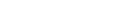Now, if we divide this N by 7, it will go evenly into the first term, and also have a remainder of 3.  In this case, the quotient when dividing by 7 would be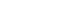because that’s exactly how many times 7 would go into that first term above.  This doesn’t obey the condition in our problem, because the second quotient is supposed to be MORE than five times the first quotient.  In order to do that, we would need the first remainder to be bigger than 7, say, 23.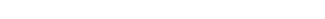I’ve written it like that to show what happens when we divide this same number by 7.  When we divide by 7, it will go five times the first quotient into that first terms, but then there are three more sevens.  Thus: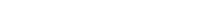In order for the second quotient to be MORE than five times the first quotient, the first quotient must have a remainder bigger than 7, so that 7 divides into it at least once, thus increasing the second quotient.   Thus, the first remainder is always bigger than 7, and the second remainder, the remainder when dividing by 7, absolutely has to be less than 7.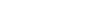That was an explanation that involving thinking about the numbers.  Here, also, is a more algebraic approach.  For more detailed information, see this GMAT blog on remainders.  We will use the “rebuilding the dividend equation” from that blog; this can be a handy equation to know.  This is the equation:

N = D*Q + R

N is the original number divided, known as the “dividend.”  D is the divisor, the number by which we divided.  Q is the integer quotient, how many times the whole of D goes into N.  R is the remainder.   Here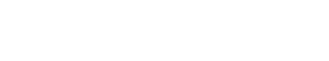Save that equation.  Now, go to the inequality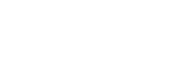Notice that the left side of this inequality is equal to the left side of the equation above.  Substitute the right side of the equation into the inequality: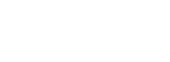9) This is a HARD problem, probably harder than anything that conceivably could appear on the GRE.  First of all, you should appreciate the significance of Quantity A.  If J is a prime number, it would have two factors.  If J is the square of a prime number, it would have exactly three factors.  A product of two primes has four factors, and products of non-primes have many more factors.

We notice that for N = 0, J = 41, a prime number, and for N = 1, J = 43, another prime number.  Clearly, in those two cases, the number of factors of J is two, less than 4, and Quantity B is bigger.  The question is: will J always be prime?

Well, if you rely only on plugging numbers, this problem is particular devious.  You see, this particular polynomial expression was discovered by the great mathematician Leonard Euler in 1772, and for all integer values from N = 0 to N = 39, this gives prime values of J.  You could plug in a lot of numbers, and get a prime number every time.  But, of course, plug-in alone does not establish a pattern.

Here’s the big idea that could help you on the GRE: It is mathematically impossible to produce any algebraic rule that consistently outputs nothing but prime numbers.  The pattern of prime numbers is the hardest pattern in mathematics to map.  No single formula will do the job.  No single formula will always, always, always give us prime numbers without exception: that is absolutely impossible.  This particular formula is famous because it happens to give a lot of prime numbers at the beginning, but some of those values will not be prime numbers.  For example, when N = 41, J = 41*41 + 41 + 41 = 43*41, which has four factors.  There is not a consistent pattern.

•